# ANSYS函数边界加载$P = 0.441 \times \left| {\sin (\omega t)} \right| \times (0.5 \times {\cos ^2}\phi \times \cos \theta – {\sin ^2}\phi )$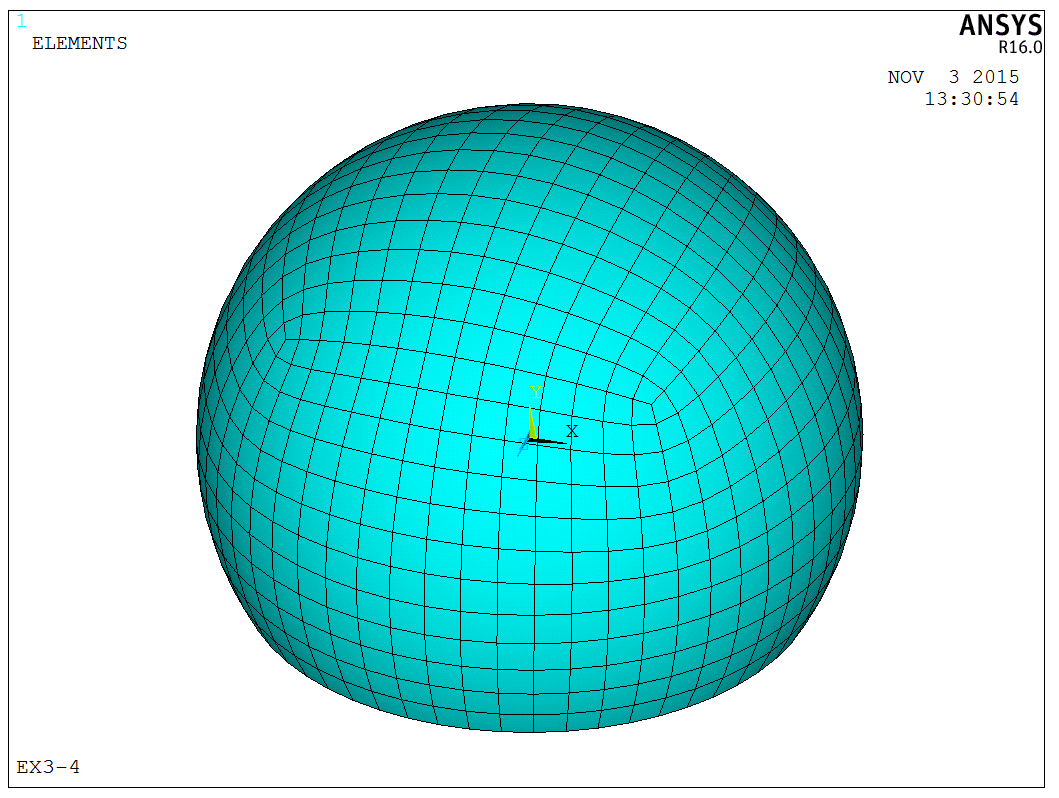P=0.441*abs(sin(w*{TIME}))*(0.5*(cos({Z}))^2*cos({Y})-(sin({Z}))^2)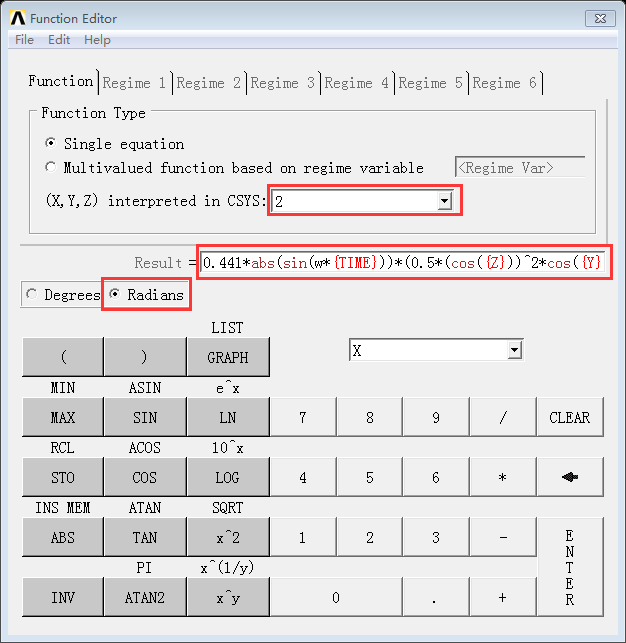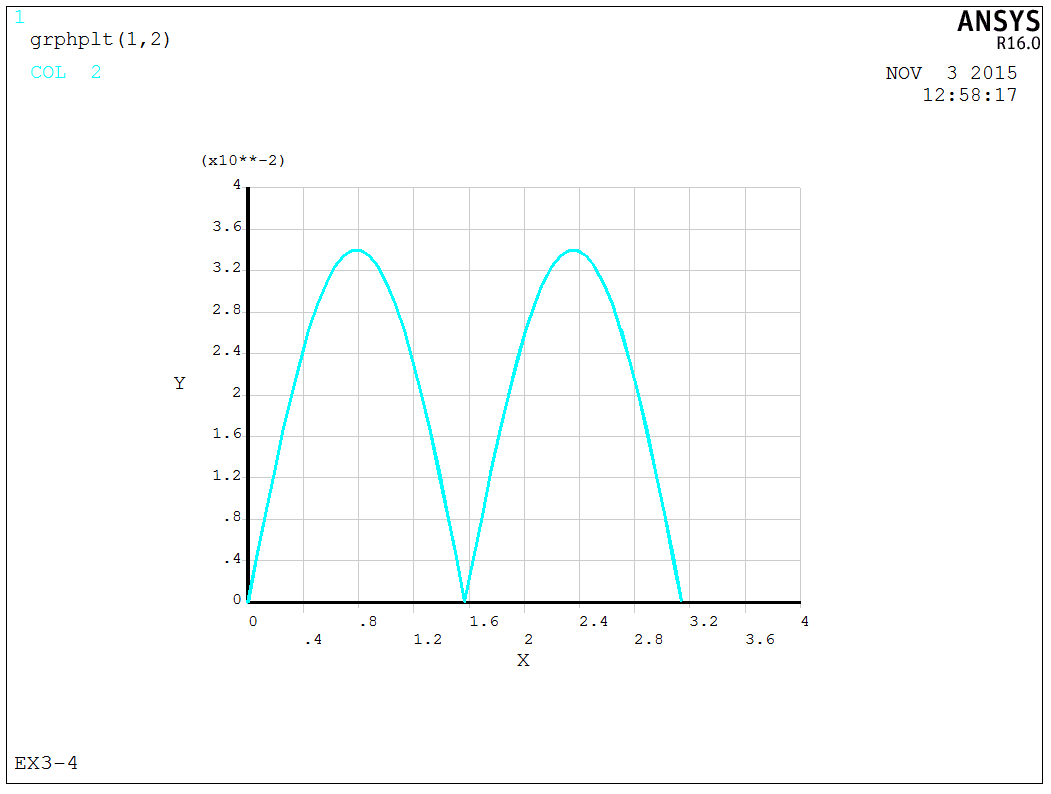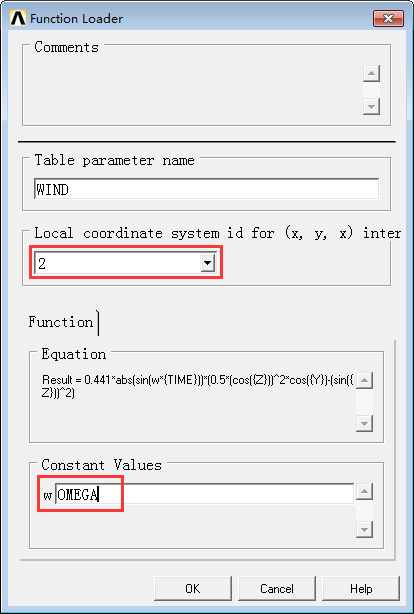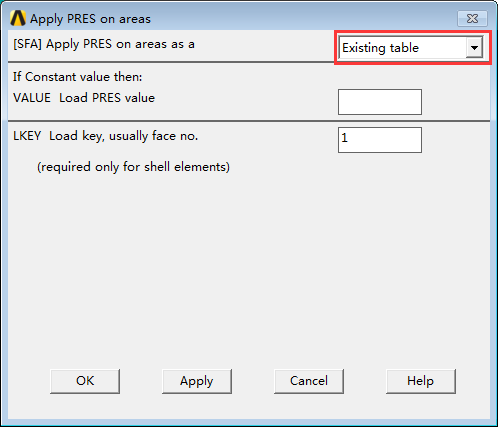TABLE加载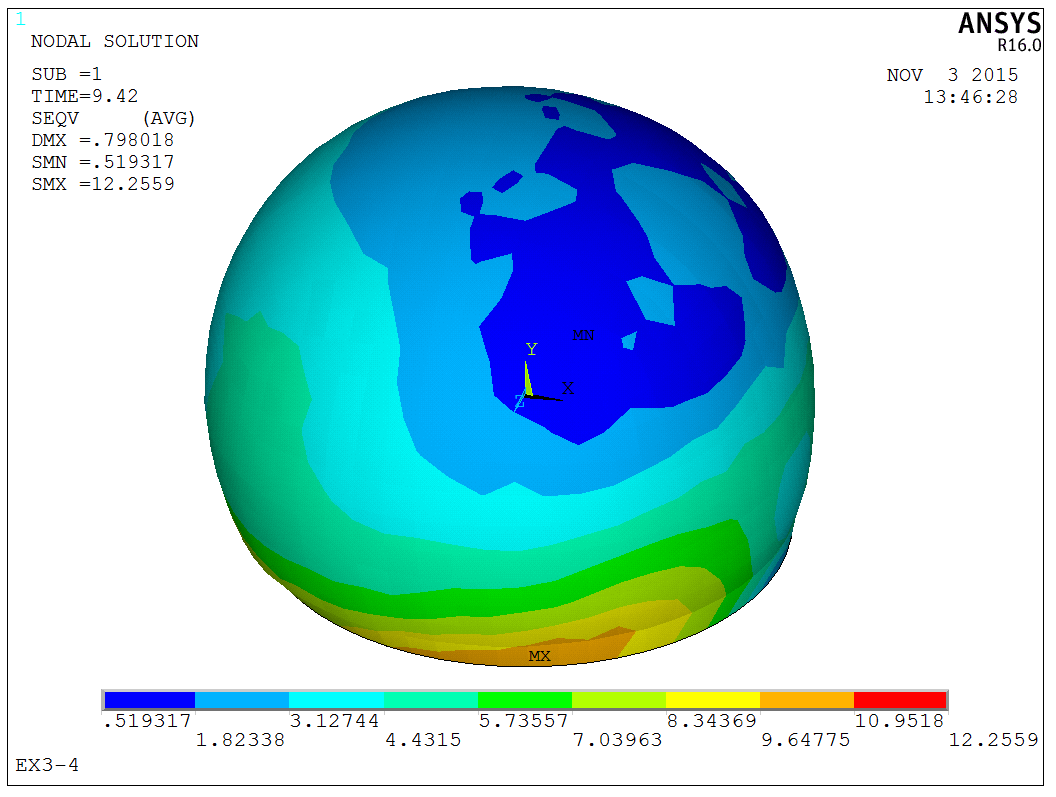X方向弯矩图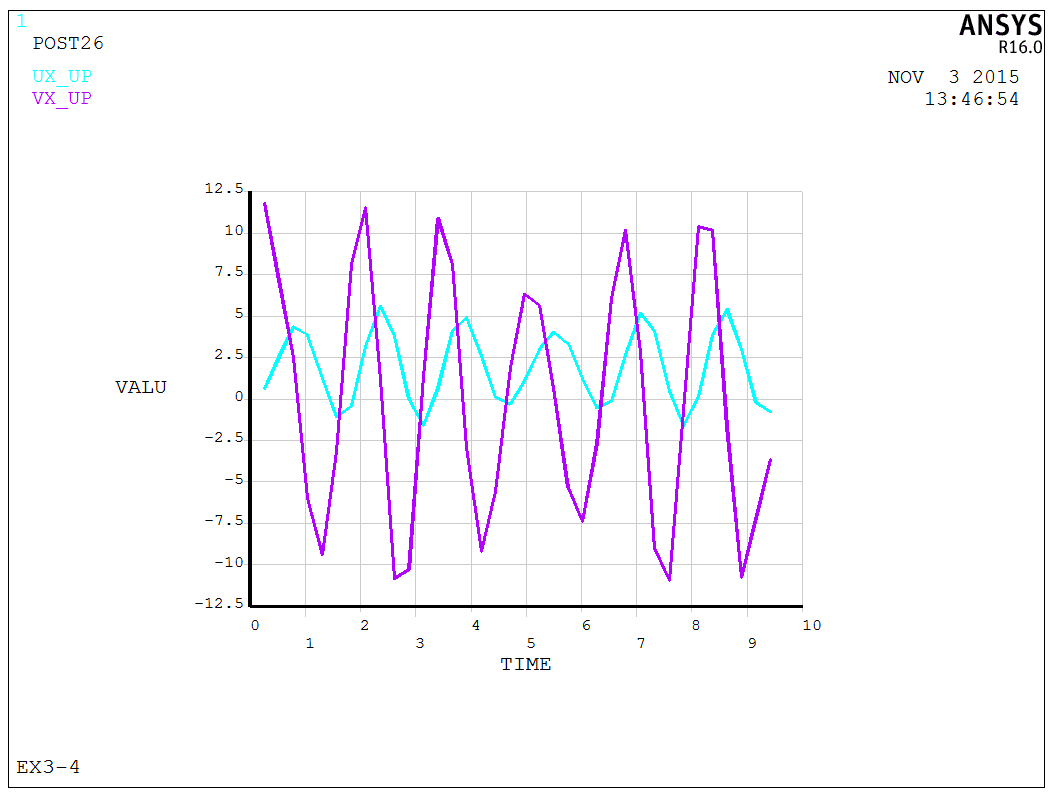X向位移随时间变化曲线

APDL代码以及相应的WIND函数文件如下所示：### 2条评论

1.酷特尔   •

完全看不懂！表示我是屌丝一枚！啊哈哈~

•伊犁的秋天   •     Author

专业不同，划划水~~~